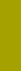﻿ LogarithmSand Texture Sedimentology | Variables

Logarithm

Logarithms of independent variables

Logarithm gives a relative scaling to a variable. Without this scaling, the variable changes are linear and independent of any value. This is in conflict with physical nature of the variables in this paper. For example, linear change of grain size, say ±1 millimeter around a mean size of 1 millimeter, dramatically distorts particularity the material finer than 2 millimeters: the "coarse" interval, 1mm - 2mm, includes a coarse sand class only, whereas the "fine" interval, 0mm - 1mm, contains every­thing finer than 1mm without a fine limit: not only a medium and fine sand, silt, clay, and colloidal classes, but also molecular, atomic and subatomic sizes approaching indefinitely zero. The same change of ±1 millimeter loses completely a physical (and common) sense for mean size smaller than 1 millimeter because zero and negative size does not exist.

Logarithmic notation also solves the zero value problem: whereas zero value of a quantity, such as an object's size makes that quantity (and object itself) non-existent, still some "scientific" literature uses zero values in diagram scales and in mathematical operations with those quantities (such as using normal size distribution instead of log normal size distribution). Logarithmic notation eliminates the zero from consideration in a natural way: any logarithm of zero is minus infinity...

Logarithmic scaling has long been applied unconsciously and intuitively as a common sense solution by introducing geometric progressions and scales (Archimedes, 287 - 212 b.C.). In astronomy about 130 BC, Hipparchus applied a geometric progression for brightness classification of stars.

Norman Pogson (1856) has adjusted the Hipparchus' “six magnitude classes” to the actually measured brightness (intensity). He defined these two scales by the following relationship: magnitude = -lognbrightness = -n x logBRI. This reads “magnitude equals to the negative logarithm (to the base n) of the brightness” where n is the base of the logarithm and equals to the brightness (intensity) ratio for a magnitude difference of 1: n = 1000.2 = 1001/5 = 2.511886432. Conversely, brightness = nmagnitude = 100MAG/5. Therefore, a magnitude difference of 5 corresponds to a brightness ratio of 100; a magnitude difference of 1 corresponds to a brightness ratio of n = 1000.2 = 100(1/5) = 2.511886432. To divide the visible stars into six brightness values was no personal idiosyncrasy of Hipparchus. It was due, although he did not realize it, to an in-built human physical mechanism of perception which operates on any change of stimulus on a logarithmic scale (the Weber - Fechner law).

In architecture, the golden section ratio controlled proportions first from esthetic reasons, but soon also from practical constructional advantages. Acoustic perception of doubled frequencies (halved wavelength) is known as octave interval which is a logarithm to the base 2 of tone frequency (one of the basic consonance principles in western musical harmony). In electro acoustics, telecommunication and electronics, various logarithms are defined, such as neper, decibel, decilog. Engineering notations are of logarithmic nature (e.g., electron radius being 2.82 x 10-13 cm). Geologic time was also recognized to show equally significant changes in a logarithmic scale (G. F. PHILLIPS, 1958; Nature, v. 181, No. 4624, p. 1652-3).

The first geometric (in effect logarithmic) scale important for grain size was suggested by Julius von SPARRE (1858) and Peter von RITTINGER (1862, 1863, 1867): it requires that sieve intervals correspond to grains with doubled settling velocity. Apparently, using Stokes' law, von SPARRE arrived at a scale with a quotient 20.5 (page 376). Though one may object that the Newton's not Stokes' law was valid for his grain sizes (0.125" - 8"), he surely presented a pioneer idea for a logarithmic settling velocity.

In the U.S.A., Johann August UDDEN (1898) suggested the first geometric grain size scale for soil and sediment classification: its basic value was 1 mm, and coefficient was 2 (or ½). UDDEN (1914) extended it from 12 to 19 values; Chester K. WENTWORTH (1922) made it popular, and in 1933, justified the scale grades physically.

William Christian KRUMBEIN (1934, 1936) perfected this concept into the first truly logarithmic scale of grain size, PHI, based on binary logarithm (logarithm to the base of 2). Though KRUMBEIN (1937) also introduced a scale based on decadic logarithm, ZETA (logarithm to a base of 10), and V. P. BATURIN (1943) suggested another decadic log-scale, GAMMA-scale (Pierre WEYDERT, 1976, ignorant of Baturin's GAMMA scale, called it ALPHA scale), most geologists are using the PHI scale.

Jiri BREZINA (1963) introduced the logarithmic scaling to settling rate: his BETA was a decadic logarithm of settling rate in millimeter/second.

Recognizing the need for a more suitable logarithmic scaling, Gerald V. MIDDLETON (1967) created his PSI unit in a way similar to the widely used PHI. He used the following features:

1. negative binary logarithm,
2. settling rate in cm/sec,
3. Greek letter ψ transcribed to PSI (similar to φ transcribed to PHI).

Since 1984, BREZINA extended the log scaling to include grain density, shape, and Reynolds number in order to simplify formulas and computation, however, without attempt to recommend the new logarithmic variables for common usage.

The Macrogranometer™ directly measures the distribution of local PSI settling rate; from this, distributions of other independent variables can be calculated by our SedVar™ program. All independent variables are expressed in logarithm and resolved into 401 steps. Two logarithm types are used:

1     negative binary logarithm = -logarithm to the base 2, each step is 0.02 wide:

PSI (logarithmic settling velocity, available in three versions), PHI (logarithmic grain size), LRS (logarithmic grain density) and LSF (logarithmic Shape Factor); each step is 0.02 wide.

2     positive decadic logarithm = +logarithm to the base 10, each step is 0.01 wide:

LRE (logarithmic Reynolds number, three versions are available).

 On these pages, the new logarithmic variables are expressed mostly in their non-logarithmic form for convenience. For example, the hydrodynamic Shape Factor SF' is used instead of LSF, particle density RS or Rs instead of LRS. However, the logarithmic form is implied in all considerations.